• 从文件里读取数组坐标，来做迷宫图，和起点终点坐标，运用回溯法找到所有路径，选其中步数最少输出。 程序运行成功。c语言
• ## 最短路径应用题

千次阅读 2017-01-11 03:46:58
最短路径应用Description在沙漠中有N个城邦国家（编号0~N-1），每天都有商队从本国出发将本国商品运到其它各个国家，到达各个目的国家后又将该国商品运回本国。在前往目的国家路程中，商队可能要需要从其它...
最短路径应用题

Description

在沙漠中有N个城邦国家（编号0~N-1），每天都有商队从本国出发将本国商品运到其它各个国家，到达各个目的国家后又将该国的商品运回本国。在前往目的国家的路程中，商队可能要需要从其它国家境内穿过。每穿过一个国家商队就需要获得一张该国的通关卡，以便该商队当天沿原路返回时使用。经过多年的摸索，每支商队都已经掌握了国家间的最佳线路（距离最短的路径，不计算在国家内穿过的距离）。

为了减少商队的负担，各国之间的商队做了如下约定：每天两国之间只由一国的商队负责往返运输，即：如果当天有A国的商队从A国出发去往B国，到达B国后再返回A国，那么当天就不会有B国的商队去往A国。

特别的，在一个国家境内穿过所需持有的通关卡每天都需要提前准备（因为要标明日期）。而两个国家之间的最佳路径（距离最短的路径）可能有多条。虽然商队只会选择其中的一条，但是各条最佳路径上需要经过的国家的管理者们并不知道商队会选择哪条路径，因此他们都假设商队当天会选择自己在的这条路径，都会提前准备通关卡（虽然这些通关卡最后可能并没有用上）。

请计算各个国家每天需要提前准备多少张通关卡。

注意事项：
1. 商队沿着某条最短路径从A国去往B国时，只有沿途经过的国家才需要通关卡，起点A国和终点B国都不需要通关卡。
2.  两国间的最短路径可能有多条，根据题目假设，相当于每一条路径都需要统计。
3. 每天两国之间只会有一国的商队往返，当天A国商队去往B国的路程中会领取通关卡，但是从B国返回A国的路程中不需要再领取通关卡，因此不要重复计算。
4. 商队当天是沿原路返回。
5. 商队都足够聪明不会沿着某条路转一圈又回到了本国，也即一个顶点到自身的距离为0，不存在从自身出发并指向自身的环形路径。

示例：如下图所示的有四个国家示意图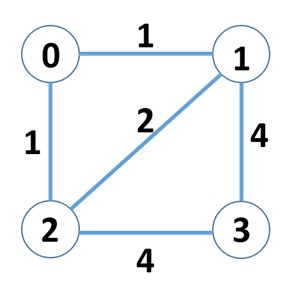国家0和1之间的最短路径为：0 → 1，没有经过除起点终点外的国家
国家0和2之间的最短路径为：0 → 2，没有经过除起点终点外的国家
国家0和3之间的最短路径有两条，分别为：0 → 1 → 3和0 → 2 → 3，分别经过国家1和国家2
国家1和2之间的最短路径有两条，分别为：1 → 2和1 → 0 → 2，其中第二条最短路径经过国家0
国家1和3之间的最短路径为：1 → 3，没有经过除起点终点外的国家
国家2和3之间的最短路径为：2 → 3，没有经过除起点终点外的国家

所以最终各个国家需要准备的通关卡依次为：1，1，1，0。国家0需要准备1张，因为国家1和2之间的其中一条最短路径经过它；国家1需要准备1张，因为国家0和3之间的其中一条最短路径经过它；同理，国家2需要准备1张；国家3不需要准备（0张），因为没有两个国家间的最短路径经过它。

Input Description

第一行为一个正整数N，表示国家的个数。国家的编号从0到N-1。N <= 400。

第二行为一个正整数E，表示国家间联通的道路。

接下来的E行，每行由三个整数组成（三个整数均在int型的表数范围内），前两个整数表示国家编号，第三个整数表示两国家间路径的距离，两个整数之间用一个空格分隔。如：1 2 6，表示国家1与国家2之间有路径相连，长度为6。这E行之外的国家间认为没有直接相连的路径。

Output Description

按照所需准备的通关卡数目从多到少的顺序进行输出，对于数目相同的国家按照编号顺序（小到大）输出。 每行输出两个整数，分别为国家编号和该国需要准备的通关卡，两个整数之间用一个空格分隔，每一行尾有换行符，最后一行也要有换行符。有几个国家就输出几行。

Input Sample

4
5
0 1 1
0 2 1
1 2 2
1 3 4
2 3 4

Output Sample

0 1
1 1
2 1
3 0

Idea

和传统Floyd算法区别在于用i->k+k->j更新i->j时，k要用一个数组保存下来。重点在于判断一个点是不是在某条最短路径上。

Code

#include<iostream>
#include<algorithm>
using namespace std;

struct Graph{
int V;
int *w;
};
struct Output{
int country;
int card_num;
};
void initGraph(Graph &G,int N){
G.V=N;
G.w=new int[N*N];
for(int i=0;i<N;i++)
for(int j=0;j<N;j++)
G.w[i*N+j]=1000;
}
void insert(Graph &G,int start,int end,int w,int *&path_num){
if(G.w[start*G.V+end]==1000){
G.w[start*G.V+end]=G.w[end*G.V+start]=w;
path_num[start*G.V+end]=path_num[end*G.V+start]=1;
}
}
void Floyd(Graph &G,int *&path_num){
for(int s=0;s<G.V;s++){
G.w[s*G.V+s]=0;
}
for(int i=0;i<G.V;i++)
for(int s=0;s<G.V;s++)
for(int e=s+1;e<G.V;e++){
if(G.w[s*G.V+e]>G.w[s*G.V+i]+G.w[i*G.V+e]){
G.w[s*G.V+e]=G.w[e*G.V+s]=G.w[s*G.V+i]+G.w[i*G.V+e];
path_num[s*G.V+e]=path_num[e*G.V+s]=path_num[s*G.V+i]*path_num[i*G.V+e];
}
else if(G.w[s*G.V+e]==G.w[s*G.V+i]+G.w[i*G.V+e]
&& i!=s && i!=e){
path_num[s*G.V+e]+=path_num[s*G.V+i]*path_num[i*G.V+e];
path_num[e*G.V+s]=path_num[s*G.V+e];
}
}
}
void Count(Graph &G,int s,int e,Output *&card,int *&path_num){
for(int i=0;i<G.V;i++){
if(G.w[s*G.V+i]+G.w[i*G.V+e]==G.w[s*G.V+e])
card[i].card_num+=path_num[s*G.V+i]*path_num[i*G.V+e];
}
}
bool cmp(Output x,Output y){
if(x.card_num!=y.card_num)
return x.card_num>y.card_num;
if(x.country!=y.country)
return x.country<y.country;
}
int main(){
int N,E,start,end,w;
cin>>N;
cin>>E;
Graph G;
initGraph(G,N);
int *path_num=new int[N*N]();
for(int i=0;i<E;i++){
cin>>start>>end>>w;
insert(G,start,end,w,path_num);
}
Output *card=new Output[N];
for(int i=0;i<N;i++){
card[i].country=i;
card[i].card_num=0;
}
Floyd(G,path_num);
for(int s=0;s<G.V-1;s++)
for(int e=s+1;e<G.V;e++)
Count(G,s,e,card,path_num);
sort(card,card+N,cmp);
for(int i=0;i<N;i++)
cout<<card[i].country<<" "<<card[i].card_num<<endl;
delete[] card;
delete[] path_num;
delete[] G.w;

return 0;
}
展开全文• Frogger最短路径典型题目图解胜于一切苍白文字思路Dijkstra（迪杰斯特拉算法）Bellman-Ford（贝尔曼-福特算法）Floyd（弗洛伊德算法） 题目 大意是求一条通路中所有相邻两个结点最大值，该最大值又是所有通路...
Frogger最短路径典型题题目图解胜于一切苍白的文字思路Dijkstra（迪杰斯特拉算法）Bellman-Ford（贝尔曼-福特算法）Floyd（弗洛伊德算法）
题目
大意是求一条通路中所有相邻两个结点的最大值，该最大值又是所有通路中的最小值。上图一目了然。
题目链接： link.
图解胜于一切苍白的文字
图片: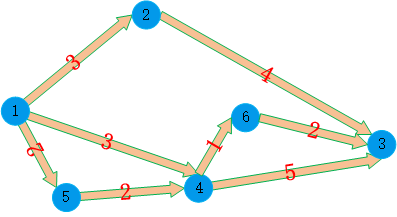结点1通往结点3的路径有：

1->2->3相邻两点间最大距离是 4 ；
1->4->3相邻两点间最大距离是 5；
1->4->6->3相邻两点间最大距离是 3；
1->5->4->3相邻两点间最大距离是 5；
1->5->4->6->3相邻两点间最大距离是 2；
故所有通路中所有相邻两个结点最大值的最小值是 通路5中：2

思路
这道题是变相的求源节点 **（本题为结点1）**到任意结点的所有路径上相邻两结点的最大距离的最小值。即动态规划的最有子解。
可以利用求最短路径的方法求解该问题。
Dijkstra（迪杰斯特拉算法）
#include<iostream>
#include<string>
#include<vector>
#include<set>
#include<algorithm>
#include<iomanip>
#include<cmath>
using namespace std;
const int MAX = 0x3f3f3f3f;
double Dist(vector<int> const& a, vector<int> const& b) {
double t;
t = sqrt(pow((double)(a - b), 2) + pow((double)(a - b), 2));
return t;
}
int main() {
int n;
int inc(0);
while (cin >> n && n) {
vector<double> dist(n + 1, MAX);
vector<bool> visited(n + 1, false);
vector<vector<int> > vec(n + 1, vector<int>(2, 0));
for (int i = 1; i <= n; i++) {
cin >> vec[i] >> vec[i];
}
dist = 0;
for (int i = 2; i <= n; i++) {
dist[i] = Dist(vec, vec[i]);
}
visited = true;
int u(1);
double maxmin(-1);
for (int m = 0; m < n;m++) {
double mindist = MAX;
for (int i = 2; i <= n; i++) {
if ((!visited[i]) && mindist > dist[i]) {
mindist = dist[i];
u = i;
}
}
visited[u] = true;
for (int j = 2; j <= n; j++) {
if (!visited[j]) {
dist[j] = min(dist[j], max( Dist(vec[u], vec[j]),dist[u]));
}
}
}
cout << fixed << setprecision(3);
cout << "Scenario #" << ++inc << endl;
cout << "Frog Distance = " << dist << endl;
cout << endl;
}

system("pause");
return 0;
}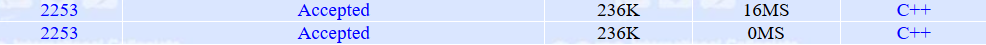Bellman-Ford（贝尔曼-福特算法）
#include<iostream>
#include<string>
#include<vector>
#include<set>
#include<algorithm>
#include<iomanip>
#include<cmath>
using namespace std;
const int MAX = 0x3f3f3f3f;
double Dist(vector<int> const& a, vector<int> const& b) {
double t;
t = sqrt(pow((double)(a - b), 2) + pow((double)(a - b), 2));
return t;
}
void relax(vector<vector<double> >& edge, int i, int j, vector<double> &dist) {
if (dist[j] > max(dist[i], edge[i][j]))
dist[j] = max(dist[i], edge[i][j]);
}
int main() {
int n;
int inc(0);
while (cin >> n && n) {
vector<double> dist(n + 1, MAX);
vector<vector<double> > edge(n+1,vector<double>(n+1));
vector<vector<int> > vec(n + 1, vector<int>(2, 0));
for (int i = 1; i <= n; i++) {
cin >> vec[i] >> vec[i];
}
dist = 0;
for (int i = 1; i <= n; i++) {
for(int j=1;j<=n;j++)
edge[i][j] = Dist(vec[i], vec[j]);
}
for (int i = 1; i <= n; i++) {
for (int s = 1; s <= n; s++) {
for (int t = 1; t <= n; t++) {
relax(edge, s, t,dist);
}
}
}

cout << fixed << setprecision(3);
cout << "Scenario #" << ++inc << endl;
cout << "Frog Distance = " << dist << endl;
cout << endl;
}

system("pause");
return 0;
}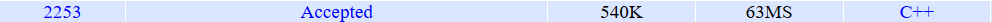Floyd（弗洛伊德算法）
#include<iostream>
#include<string>
#include<vector>
#include<set>
#include<algorithm>
#include<iomanip>
#include<cmath>
using namespace std;
const int MAX = 0x3f3f3f3f;
double Dist(vector<int> const& a, vector<int> const& b) {
double t;
t = sqrt(pow((double)(a - b), 2) + pow((double)(a - b), 2));
return t;
}
int main() {
int n;
int inc(0);
while (cin >> n && n) {
vector<vector<double> > dist(n + 1, vector<double>(n+1,MAX));
vector<bool> visited(n + 1, false);
vector<vector<int> > vec(n + 1, vector<int>(2, 0));
for (int i = 1; i <= n; i++) {
cin >> vec[i] >> vec[i];
}
for (int i = 1; i <= n; i++) {
for(int j=1;j<=n;j++)
dist[i][j] = Dist(vec[i], vec[j]);
}
for (int k = 1; k <= n; k++) {
for (int i = 1; i <= n; i++) {
if (i == k) continue;
for (int j = 1; j <= n; j++) {
if (j == k) continue;
if (dist[i][j] > max(dist[i][k], dist[k][j])) {
dist[i][j] = max(dist[i][k], dist[k][j]);
}
}
}
}

cout << fixed << setprecision(3);
cout << "Scenario #" << ++inc << endl;
cout << "Frog Distance = " << dist << endl;
cout << endl;
}

system("pause");
return 0;
}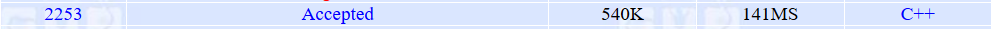展开全文c++ 后端 数据结构
• 单源最短路径——Dijkstra 时间复杂度：O(n^2) 贪心算法 当图中存在负权值时不适用，改用Folyd for循环 { 选出最近点，把该点加入集合 计算从该点出发到其他所有点距离（排除集合中点）并更新距离 } #include&...
单源最短路径——Dijkstra
时间复杂度：O(n^2)
贪心算法
当图中存在负权值时不适用，改用Folyd
for循环
{
选出最近的点，把该点加入集合
计算从该点出发到其他所有点的距离（排除集合中的点）并更新距离
}
#include<bits/stdc++.h>
using namespace std;
#define inf 0x3f3f3f

int n, s, e; //n为点的个数，s，e分别为起点终点
int mmap; //各点之间的距离，若为无向图则对称
int dis, sset = {0}; //dis为到原点的距离，sset为该点是否加入集合
vector<int> path;  //path为各点到原点的最短路径

void dij(){
for(int i=0; i<n; i++){
dis[i] = inf;
}
dis[s] = 0;
path[s].push_back(s);
for(int i=0; i<n; i++){
int mmin = inf;
int now;
for(int j=0; j<n; j++){
if(dis[j] < mmin && sset[j] == 0){
mmin = dis[j];
now = j;
}
}
sset[now] = 1;
for(int j=0; j<n; j++){
if(sset[j] == 0 && (dis[now] + mmap[now][j] < dis[j])) {
dis[j] = dis[now] + mmap[now][j];
path[j] = path[now];
path[j].push_back(j);
}
}
}
}

int main(){
cin >> n;
for(int i=0; i<n; i++)
for(int j=0; j<n; j++)
mmap[i][j] = inf;
for(int i=0; i<n; i++)
for(int j=0; j<n; j++){
cin >> mmap[i][j];
if(mmap[i][j] == 100)
mmap[i][j] = inf;
}

cin >> s >> e;
dij();
cout << dis[e] << endl;
for(int i=0; i<path[e].size() - 1; i++)
cout << path[e][i] << "->";
cout << path[e][path[e].size() - 1] << endl;
}

全对最短路径——Floyd
时间复杂度: O(n^3)
贪心算法
适用于图中含有正、负权值
步骤：
1⃣️初始化map矩阵
矩阵中map[i][j]的距离为顶点i到顶点j的权值；
如果i和j不相邻，则map[i][j]=∞。
如果i==j，则map[i][j]=0;
2⃣️以顶点A(假设是第k个顶点)为中介点，若a[i][k]+a[k][j] < a[i][j]，则设置a[i][j]=a[i]+a[j]。
#include<bits/stdc++.h>
using namespace std;
#define inf 0x3f3f3f

int n, s, e; //n为点的个数，s，e分别为起点终点
int mmap; //各点之间的距离，若为无向图则对称
int path; //path为i,j之间的点

void floyd(){
for(int i=0; i<n; i++)
for(int j=0; j<n; j++){
path[i][j] = -1;
}
for(int k=0; k<n; k++){
for(int i=0; i<n; i++){
for(int j=0; j<n; j++){
if(mmap[i][k] + mmap[k][j] < mmap[i][j]){
mmap[i][j] = mmap[i][k] + mmap[k][j];
path[i][j] = k;
}
}
}
}
}

int main(){
cin >> n;
for(int i=0; i<n; i++)
for(int j=0; j<n; j++)
mmap[i][j] = inf;
for(int i=0; i<n; i++)
for(int j=0; j<n; j++){
cin >> mmap[i][j];
if(mmap[i][j] == 100)
mmap[i][j] = inf;
}

cin >> s >> e;
floyd();
cout << mmap[s][e] << endl;
cout << s << " ";
vector<int> dis;
int k = path[s][e];
while(k != -1){
dis.push_back(k);
k = path[s][k];
}
for(int i=dis.size()-1; i>=0; i--)
cout << dis[i] << " ";
cout << e << endl;
}

SPFA
可以解决带有负权边，负环的问题
例题可参考

https://blog.csdn.net/pursue_my_life/article/details/80201499

初始化代码
void init()
{
memset(vis, false, sizeof(vis));  //将vis[]数组置为0
memset(money, false, sizeof(money));  //将money[]数组置为0
for(int i = 0; i < maxn; ++i)
memset(cost[i], false, sizeof(cost[i]));  //将cost[][]二维数组置为0
fill( dis, dis+maxn, inf);  //将dis数组置为inf
}

memset和fill的区别与使用方法
memset：按照字节填充某字符
#include <cstring>
const int INF = 0x3f3f3f3f;
memset(a,INF,sizeof(a));

fill：按照单元赋值，将一个区间的元素都赋予val值
#include <algorithm>
fill(vec.begin(), vec.end(), val); //容器vector
fill(dis, dis+maxn, inf); //一维数组

因为memset函数按照字节填充，所以一般memset只能用来填充char型数组，（因为只有char型占一个字节）。如果填充int型数组，只能填充0、-1 和 inf（正负都行）。因为00000000 = 0，-1同理，如果我们把每一位都填充“1”，会导致变成填充入“11111111”。如果我们将inf设为0x3f3f3f3f，0x3f3f3f3f的每个字节都是0x3f！所以要把一段内存全部置为无穷大，我们只需要memset(a,0x3f,sizeof(a))。无穷小可以将-INF设为0x8f。
而fill函数可以赋值任何值
memset比fill处理速度快一些，所以在能满足需要时，推荐用memset


展开全文算法 dijkstra c++
• 13.4 课题学习 最短路径问题;知识点最短路线问题 1如图小明到小丽家有四条路其中路程最短是( ) A B C D;精品资料; 你怎么称呼老师 如果老师最后没有总结一节课重点难点你是否会认为老师教学方法需要改进 你...
• 一顶点到其余各顶点;一单源最短路径 (Dijkstra算法(1;3;4;5;6;7;顶点A到其他顶点的最短路径(v0,v2)+ (v2,v3(v0,v3;...6已知带权有向图如图3所示请利用Dijkstra 算法从顶点V4出发到其余顶点的最短路径及长度给出相应
• 文章目录Floyd算法题目提示代码Dijkstra算法题目提示代码Spfa算法题目提示代码1代码2 ...于是小Hi便开心道：“这次要说算法叫做Floyd算法，是一种用于求图结构上任意两点间最短距离算法！” 小
文章目录Floyd算法题目提示代码Dijkstra算法题目提示代码Spfa算法题目提示代码1代码2
Floyd算法
题目
HihoCoder-1089
vjudge
提示
小Ho道：“你说的很有道理，我只需要从每个节点开始使用Dijstra算法就可以了！”
小Hi摇摇头道：“解决问题不是关键，学到知识才是关键，而且知识本身也远远没有掌握学习的方法重要！”
小Ho只得答道：“好的好的，听你说便是了!”
于是小Hi便开心道：“这次要说的算法叫做Floyd算法，是一种用于求图结构上任意两点间最短距离的算法！”
小Ho嘀咕道：“你都写标题上了，能不知道么？”
小Hi强行装作没听到，继续说道：“这个算法的核心之处在于数学归纳法——MinDistance(i, j)之间最短路径中可以用到的节点是一点点增加的！”
“你这话每一个字我都听得懂，但是这句话为什么我听不懂呢……”小Ho无奈道。
“那我这么说吧，首先，最开始的时候，MinDistance(i, j)——即从第i个点到第j个点的最短路径的长度，拥有一个限制：这条路径不能经过任何节点。”小Hi道。
“那就是说如果从i个点到第j个点之间没有直接相连的边的话，这个长度就是无穷大咯？”小Ho总结道：“只需要把输入的边填进MinDistance中即可！”
“对！”小Hi满意于小Ho的上道，继续说道：“然后我放开限制，我允许MinDistance(i, j)——从第i个点到第j个点的最短路径的长度，拥有的限制，变为：这条路径仅允许经过1号节点。”
“这个也简单，对于两个节点i, j，我只需要比较MinDistance(i, j)原来的值和MinDistance(i, 1)+MinDistance(1, j)的值，取较小的一个作为新的MinDistance(i, j)就可以了——毕竟原来的MinDistance都是不经过任何节点，那么这样求出来的新的MinDistance(i, j)只有可能经过1号节点。”
“那么接下来就是关键的了，我将限制继续放宽——路径仅允许经过1、2号节点。”小Hi继续说道。
“那其实也没有任何变化吧，对于两个节点i, j，我只需要比较MinDistance(i, j)原来的值和MinDistance(i, 2)+MinDistance(2, j)的值，取较小的一个作为新的MinDistance(i, j)，之所以可以这样是因为，原来的MinDistance都是在限制“仅允许经过1号节点”下，求出来的，所以新求出来的MinDistance(i, j)也只有可能经过1、2号节点！“
“那我继续放开限制呢？”小Hi问道。
“也没有什么区别了，每放开一个新的节点k允许作为路径中的节点，就对于任意的i, j，用MinDistance(i, k)+MinDistance(k, j)去更新MinDistance(i, j)，直到1…N号节点都被添加进限制，此时也就等于没有限制了，那么这个时候的MinDistance(i, j)就是我们所想要求的值，写成伪代码就是这样！”
for k = 1 .. N
for i = 1 .. N
for j = 1 .. N
若i, j, k各不相同
MinDistance[i, j] = min{MinDistance[i, j], MinDistance[i, k] + MinDistance[k, j]}

“看来你已经很明白了呢！”小Hi嘿嘿一笑，往鬼屋深处跑了去——那么接下来就是小Ho利用求出的最短路径，去找到小Hi的时候了！
代码
#pragma GCC optimize(3,"Ofast","inline")
#pragma G++ optimize(3,"Ofast","inline")

#include <iostream>
#include <cstdio>
#include <cmath>
#include <cstring>
#include <algorithm>

#define RI                 register int
#define re(i,a,b)          for(RI i=a; i<=b; i++)
#define ms(i,a)            memset(a,i,sizeof(a))
#define MAX(a,b)           (((a)>(b)) ? (a):(b))
#define MIN(a,b)           (((a)<(b)) ? (a):(b))

using namespace std;

typedef long long LL;

const int N=1005;
const int inf=0x3f3f3f;

int n,m;
int f[N][N];

int main() {
scanf("%d%d",&n,&m);
memset(f,inf,sizeof(f));
for(int i=1; i<=m; i++) {
int u,v,w;
scanf("%d%d%d",&u,&v,&w);
f[u][v]=MIN(f[u][v],w);
f[v][u]=MIN(f[v][u],w);
}
for(int k=1; k<=n; k++)
for(int i=1; i<=n; i++)
for(int j=1; j<=n; j++)
f[i][j]=MIN(f[i][j],f[i][k]+f[k][j]);
for(int i=1; i<=n; i++) {
for(int j=1; j<=n; j++)
printf("%d ",(i==j) ? 0 : f[i][j]);
printf("\n");
}
return 0;
}

Dijkstra算法
题目
HihoCoder-1089
vjudge
提示
小Ho想了想说道：“唔……我觉得动态规划可以做，但是我找不到计算的顺序，如果我用f[i]表示从S到达编号为i的节点的最短距离的话，我并不能够知道f…f[N]的计算顺序。”
“所以这个问题不需要那么复杂的算法啦，我就稍微讲讲你就知道了！”小Hi道：“路的长度不可能为负数对不对？”
“那是自然，毕竟人类还没有发明时光机器……”小Ho点点头。
于是小Hi问道：“那么如果就看与S相邻的所有节点中与S最近的那一个S’，并且从S到S’的距离为L，那么有可能存在另外的道路使得从S到S’的距离小于L么？”
“不能，因为S’是与S相邻的所有节点中与S最近的节点，那么从S到其他相邻点的距离一定是不小于L的，也就是说无论接下来怎么走，回到L点时总距离一定大于L。”小Ho思考了一会，道。
“也就是说你已经知道了从S到S’的最短路径了是么？”小Hi继续问道。
“是的，这条最短路径的长度是L。”小Ho答道。
小Hi继续道：“那么现在，我们不妨将S同S’看做一个新的节点？称作S1，然后我就计算与S相邻或者与S’相邻的所有节点中，与S最近的哪一个节点S’’。注意，在这个过程中，与S相邻的节点与S的距离在上一步就已经求出来了，那么我要求的只有与S’相邻的那些节点与S的距离——这个距离等于S与S’的距离加上S’与这些结点的距离，对于其中重复的节点——同时与S和S’相邻的节点，取两条路径中的较小值。”
小Ho点了点头：“那么同之前一样，与S1（即S与S’节点）相邻的节点中与S’距离最近的节点如果是S’‘的话，并且这个距离是L2，那么我们可以知道S到S’'的最短路径的长度便是L2，因为不可能存在另外的道路比这个更短了。”
于是小Hi总结道：“接下来的问题不就很简单了么，只需要以此类推，每次将与当前集合相邻（即与当前集合中任意一个元素）的所有节点中离S最近的节点（这些距离可以通过上一次的计算结果推导而出）选出来添加到当前集合中，我就能够保证在每一个节点被添加到集合中时所计算的离S的距离是它与S之间的最短路径！”
“原来是这样！但是我的肚子更饿了呢！”言罢，小Ho的肚子咕咕叫了起来。
代码
Dijkstra：
#pragma GCC optimize(3,"Ofast","inline")
#pragma G++ optimize(3,"Ofast","inline")

#include <iostream>
#include <cstdio>
#include <cmath>
#include <cstring>
#include <algorithm>

#define RI                 register int
#define re(i,a,b)          for(RI i=a; i<=b; i++)
#define ms(i,a)            memset(a,i,sizeof(a))
#define MAX(a,b)           (((a)>(b)) ? (a):(b))
#define MIN(a,b)           (((a)<(b)) ? (a):(b))

using namespace std;

typedef long long LL;

const int N=1005;
const int inf=0x3f3f3f;

int n,m,s,t;
int d[N],vis[N];
int a[N][N];

void dijkstra() {
memset(vis,0,sizeof(vis));
for(int i=1; i<=n; i++) d[i]=inf;
d[s]=0;
for(int i=1; i<=n; i++) {
int k=-1;
for(int j=1; j<=n; j++)
if(!vis[j] && (k==-1 || d[k]>d[j])) k=j;
vis[k]=1;
for(int j=1; j<=n; j++)
if(!vis[j] && d[k]+a[k][j]<d[j])
d[j]=d[k]+a[k][j];
}
}

int main() {
scanf("%d%d%d%d",&n,&m,&s,&t);
memset(a,inf,sizeof(a));
for(int i=1; i<=m; i++) {
int u,v,w;
scanf("%d%d%d",&u,&v,&w);
a[u][v]=MIN(a[u][v],w);
a[v][u]=MIN(a[v][u],w);
}
dijkstra();
printf("%d\n",d[t]==inf ? -1 : d[t]);
return 0;
}


堆优化Dijkstra：
#pragma GCC optimize(3,"Ofast","inline")
#pragma G++ optimize(3,"Ofast","inline")

#include <iostream>
#include <cstdio>
#include <cmath>
#include <cstring>
#include <algorithm>
#include <queue>

#define RI                 register int
#define re(i,a,b)          for(RI i=a; i<=b; i++)
#define ms(i,a)            memset(a,i,sizeof(a))
#define MAX(a,b)           (((a)>(b)) ? (a):(b))
#define MIN(a,b)           (((a)<(b)) ? (a):(b))

using namespace std;

typedef long long LL;

const int N=1005;
const int M=10005;
const int inf=0x3f3f3f;

struct Edge {
int to,nt,w;
} e[M<<1];

struct Node {
int index,dist;

bool operator < (const Node &rhs) const {
return dist>rhs.dist;
}
};

int n,m,s,t,cnt;
int d[N],vis[N],h[N];

priority_queue<Node> q;

inline void add(int u,int v,int w) {
e[++cnt].to=v;
e[cnt].nt=h[u];
e[cnt].w=w;
h[u]=cnt;
}

void dijkstra() {
memset(vis,0,sizeof(vis));
for(int i=1; i<=n; i++) d[i]=inf;
d[s]=0;
q.push((Node){s,0});
while(!q.empty()) {
Node x=q.top();
q.pop();
int u=x.index;
if(vis[u]) continue;
vis[u]=1;
for(int i=h[u]; i; i=e[i].nt) {
int v=e[i].to;
if(d[v]>d[u]+e[i].w) {
d[v]=d[u]+e[i].w;
q.push((Node){v,d[v]});
}
}
}
}

int main() {
scanf("%d%d%d%d",&n,&m,&s,&t);
for(int i=1; i<=m; i++) {
int u,v,w;
scanf("%d%d%d",&u,&v,&w);
add(u,v,w);
add(v,u,w);
}
dijkstra();
printf("%d\n",d[t]==inf ? -1 : d[t]);
return 0;
}


Spfa算法
题目
HihoCoder-1089
vjudge
提示
“唔……地点很多，道路很少，这个鬼屋是一个稀疏图，既然这一点被特地标注出来，那么想来有其作用的咯？”小Ho道。
“是的，正好有一种最短路径算法，它的时间复杂度只和边的条数有关，所以特别适合用来解决这种边的数量很少的最短路问题！”小Hi点了点头道：“它就是SPFA算法，即Shortest Path Faster Algorithm。”
“听上去很厉害的样子，但是实际上怎么做的呢？”小Ho问道。
“你会用宽度优先搜索写这道题么？”小Hi反问道。
“这个当然会啊，构造一个队列，最开始队列里只有(S, 0)——表示当前处于点S，从点S到达该点的距离为0，然后每次从队首取出一个节点(i, L)——表示当前处于点i，从点S到达该点的距离为L，接下来遍历所有从这个节点出发的边(i, j, l)——表示i和j之间有一条长度为l的边，将(j, L+l)加入到队尾，最后看所有遍历的(T, X)节点中X的最小值就是答案咯~”小Ho对于搜索已经是熟稔于心，张口便道。
“SPFA算法呢，其实某种意义上就是宽度优先搜索的优化——如果你在尝试将(p, q)加入到队尾的时候，发现队列中已经存在一个(p, q’)了，那么你就可以比较q和q’：如果q>=q’，那么(p, q)这个节点实际上是没有继续搜索下去的必要的——算是一种最优化剪枝吧。而如果q&ltq’，那么(p, q’)也是没有必要继续搜索下去的——但是它已经存在于队列里了怎么办呢？很简单，将队列中的(p, q’)改成(p, q)就可以了！”
“那我该怎么知道队列中是不是存在一个(p, q’)呢？” <额，维护一个position[1…n]的数组就可以了，如果不在队列里就是-1，否则就是所在的位置！”< p>
“所以说这本质上就是宽度优先搜索的剪枝咯？”小Ho问道。
小Hi笑道：“怎么可能！SPFA算法其实是BELLMAN-FORD算法的一种优化版本，只不过在成型之后可以被理解成为宽度优先搜索的！这个问题，我们会在之后好好讲一讲的！”
代码1
用vector实现。
#pragma GCC optimize(3,"Ofast","inline")
#pragma G++ optimize(3,"Ofast","inline")

#include <iostream>
#include <cstdio>
#include <cmath>
#include <cstring>
#include <algorithm>
#include <queue>
#include <vector>

#define RI                 register int
#define re(i,a,b)          for(RI i=a; i<=b; i++)
#define ms(i,a)            memset(a,i,sizeof(a))
#define MAX(a,b)           (((a)>(b)) ? (a):(b))
#define MIN(a,b)           (((a)<(b)) ? (a):(b))

using namespace std;

typedef long long LL;

const int N=1e5+5;
const int inf=1e9;

int n,m,s,t;
int v[N],d[N];

vector<int> a[N],b[N];
queue<int> q;

int spfa() {
for(int i=1; i<=n; i++) d[i]=inf;
q.push(s);
v[s]=1;
d[s]=0;
while(!q.empty()) {
int x=q.front();
q.pop();
v[x]=0;
for(int i=0; i<a[x].size(); i++) {
int tp=a[x][i];
if(d[tp]>d[x]+b[x][i]) {
d[tp]=d[x]+b[x][i];
if(!v[tp]) {
q.push(tp);
v[tp]=1;
}
}
}
}
if(d[t]==inf) d[t]=-1;
return d[t];
}

int main() {
scanf("%d%d%d%d",&n,&m,&s,&t);
for(int i=1; i<=m; i++) {
int u,v,w;
scanf("%d%d%d",&u,&v,&w);
a[u].push_back(v);
b[u].push_back(w);
a[v].push_back(u);
b[v].push_back(w);
}
printf("%d\n",spfa());
return 0;
}

代码2
用链式前向星实现。
#pragma GCC optimize(3,"Ofast","inline")
#pragma G++ optimize(3,"Ofast","inline")

#include <iostream>
#include <cstdio>
#include <cmath>
#include <cstring>
#include <algorithm>
#include <queue>

#define RI                 register int
#define re(i,a,b)          for(RI i=a; i<=b; i++)
#define ms(i,a)            memset(a,i,sizeof(a))
#define MAX(a,b)           (((a)>(b)) ? (a):(b))
#define MIN(a,b)           (((a)<(b)) ? (a):(b))

using namespace std;

typedef long long LL;

const int N=1e5+5;
const int M=1e6+5;
const int inf=1e9;

struct Edge {
int to,nt,w;
} e[M<<1];

int n,m,s,t,cnt;
int v[N],d[N],h[N];

queue<int> q;

inline void add(int a,int b,int c) {
e[++cnt]=(Edge){b,h[a],c};
h[a]=cnt;
}

int spfa() {
for(int i=1; i<=n; i++) d[i]=inf;
q.push(s);
v[s]=1;
d[s]=0;
while(!q.empty()) {
int x=q.front();
q.pop();
v[x]=0;
for(int i=h[x]; i; i=e[i].nt) {
int tp=e[i].to;
if(d[tp]>d[x]+e[i].w) {
d[tp]=d[x]+e[i].w;
if(!v[tp]) {
q.push(tp);
v[tp]=1;
}
}
}
}
if(d[t]==inf) d[t]=-1;
return d[t];
}

int main() {
scanf("%d%d%d%d",&n,&m,&s,&t);
memset(h,-1,sizeof(h));
for(int i=1; i<=m; i++) {
int u,v,w;
scanf("%d%d%d",&u,&v,&w);
add(u,v,w);
add(v,u,w);
}
printf("%d\n",spfa());
return 0;
}



展开全文C++
• 更新中：最短路径 //这个还是动态规划，创造这样一个数组location make_pair(int,int) //这个由其他变形过来，就比如给一个一维数组，每个位置可以跳不同步数，问，跳 //到数组最后一位所需要步数是多少...
• 题目描述平原上，一群蜜蜂离开蜂巢采蜜，要连续采集5片花丛后归巢，...输出描述从出发到返回蜂巢最短路径的长度取整值，取整办法为舍弃小数点后面的值。示例输入200 0 200 10 200 50 200 30 200 25输出456代码import...
• ，给出一个有向图，请输出从某一点出发到所有点的最短路径长度。 输入输出格式 输入格式： 第一行包含三个整数N、M、S，分别表示点个数、有向边个数、出发点编号。 接下来M行每行包含三个整数Fi、Gi...
• 最短路径。 思路： 刚开始不知道在想什么，写特别麻烦，一直tle，简直见鬼。 后来发现问题竟然出在判断字符相等那里写超时了。。也是佩服我自己。 就是一个最短路径，题意也不难。#include <cstdi
• 单源最短路径：计算源点到其他各顶点的最短路径的长度 全局最短路径：图中任意两点的最短路径 Dijkstra、Bellman-Ford、SPFA求单源最短路径 Floyed可以求全局最短路径，但是效率比较低 SPFA算法是Bellm...
• 题目分析：这是一道典型的最短路径模版，需要注意是：使用dijkstra算法求解需要考虑有重复边问题，而使用bellman-ford算法 和 spfa算法 可以忽略这个问题。 代码如下： // Dijkstra #in...
• 题目大意：给定n*n网络，起点K，终点T，S表示蛇(最多5条，且经过S时，若蛇是活则时间+1)，数字代表钥匙编号(最多m把且必须按照顺序取)，每次移动时间+1，求集齐所有钥匙到达终点的最短时间 bfs迷宫搜索。 vis...
• 在常用的单源点最短路径算法中，迪杰斯特拉算法是最为常用的一种，是一种按照路径长度递增的次序产生最短路径的算法。 可将迪杰斯特拉算法描述如下： 在本中，读入一个有向图的带权邻接矩阵（即数组表示），...
• 习题25.1-6 O(n^3)时间内从已经计算出的最短路径权重矩阵L计算出...如此可遍历L矩阵的第i行所有元素L[i, k]，若L[i, j]= L[i, k]+ w[k, j]，表明k是i-> j最短路径的j的前驱结点。 习题25.1-7 在Extend_Shortest_Pat
• 目 最短路径算法分类与应用研究 学 院 专 业 班 级 学生姓名 指导教师 2008年10月 最短路径算法分类与应用研究 姓 名 班 级 指导教师 摘要 本文研究目的在丁收集整理关丁最短路径的普遍算法 为研究最短路径I可在一...
• 习题21.1-3 Bellman-Ford算法改进为m+1次松弛后终止。图中结点若在s->v路径中则作标记。松弛过程中，若有标记结点...用2维矩阵保存所有结点之间的最短路径，也包括自己到自己路径。然后按照Bellman-Ford算法运行
• 最近在练习PAT，遇到一个要求解最短路径的题，很自然地想起了这学期刚学的迪杰斯特拉算法。但是这个问题要求解多条最短路径，而迪杰斯特拉算法只能求出其中一条最短路径及其距离，所以要在运用迪杰斯特拉算法算法的...
• 在带权有向图G中，求G中任意一对顶点间的最短路径问题，也是十分常见一种问题。 解决这个问题一个方法是执行n次迪杰斯特拉算法，这样就可以求出每一对顶点间的最短路径，执行时间复杂度为O(n3)。 而另一种...
• 最短路 Time Limit: 5000/1000 MS (Java/Others) Memory Limit: 32768/32768 K (Java/Others) Total Submission(s): 36954 Accepted Submission(s): 16091 ...在每年校赛里，所有进入决赛同学都会获dijstra算法
• 最短路径题。大家先在草稿本上，认真地做一遍，然后再看后面视频。期待您在评论区留言。温馨提醒：因为视频内容越来越多，为了更好把内容进行分类归纳，方便大家更系统学习，将所有内容优化成三个微信公众号，...
• 是我太笨了，想不到这么巧妙方法，说出来呢其实就是迪杰斯特拉单源最短路径算法一点扩展和变种，可能没见过所以就不会做！！！！！！ 哦对，这不是我自己做，是看了大佬博客，以下是链接，自取自取：传送...dijkstra 算法
• 题解：公路和铁路都到达n时最小值，有题意可知如果铁路直接到达n公路就不能直接到达（求公路到达最小值即可），同理公路能到达铁路就不能到达（求铁路到达最小值即可）。 在一个叫奥斯汀城市，有n个小镇...
• 最短路径算法是算法竞赛中经常出现的一种算法，关于它的应用非常丰富，在这里我收集了一些我...用Dijkstra算法找最短路径，在找的同时记录最短路径的数量，以及不断更新能召集的最大救援队数量。 代码参考自https:/...
• 最短路 Time Limit: 5000/1000 MS (Java/Others) Memory Limit: 32768/32768 K (Java/Others) Total Submission(s): 33752 Accepted Submission(s): 14662 ...在每年校赛里，所有进入决赛同学都会获
• 今天也是为了cc，努力奋斗一天ヾ(≧▽≦*)o 疑问 暂无 代码 #include<stdio.h> #include<algorithm> using namespace std; //最大数量 const int MAXV = 1010; //定义不可达时距离 ...算法 c++ dijkstra
• 众所周知，迪杰斯特拉算法是求单源最短路，是一个点到多个点的最短路径 这个题目给你图是路都是单向路。 而这先让你求多个点到单个点最短路，然后再求单个点到多个点最短路。 求多个点到单个点...
• 最近在做最短路径的题的时候，经常用到SPFA，但是我不是很喜欢用STL，所以自己模拟了一下队列，但是针对HDU上大数据的题总是RE  现在明白了，队列的话，我要是开的稍微小一点的话，那么队列的开头是一直在后退，......